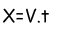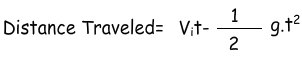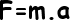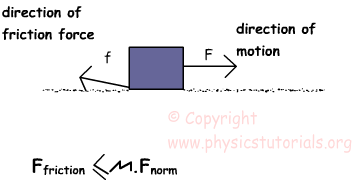Dynamics (Relative Motion, Projectile Motion Newton’s Laws) Cheat Sheet

Relative Motion: Velocity of the moving objects with respect to other moving or stationary object is called “relative velocity” and this motion is called “relative motion”. We use following equation to find relative velocity of the objects;

Vrelaltive=Vobject-Vobserver

Projectile Motion: We can say that motion in two dimensions or motion under the effect of gravitational force is called projectile motion. We can use the equations of motion given in the kinematics here. We change the acceleration with gravitational acceleration. This motion can analyzed under two title

Horizontal Motion: We find velocity and distance with the formula;Vertical Motion: We find velocity and distance with the formula;

V=Vit+gt where acceleration is -9,8m/s² and Vi=initial velocityNEWTON’S LAW OF MOTION

Newton’s First Law of Motion: If an object is at rest it continuous to be at rest and if it is moving it continuous to move unless a nonzero force is applied on it.

Newton’s Second Law of Motion: Applied nonzero force (net force) on an object gives it acceleration.Newton’s Third Law of Motion: In this law Newton states that, when we apply a force on something then it also apples force on us in same magnitude but opposite in direction.Force

What change the state of object is called “force”. We mean by saying state, shape or position of the object.

Normal Force: Contact objects exert force to each other because of their weights. These forces are equal in magnitude but opposite in direction.Fnormal=mg=weight of the book

Friction Force: Friction force results from the interactions of surfaces. Irregularities in the structure of the matters causes friction force.Friction force is always opposite to the direction of motion.We can calculate the friction force by the formula given above;

Where; µ is the coefficient of friction and Fnorm is the normal force acting on the surface.

Dynamics Exams

Related